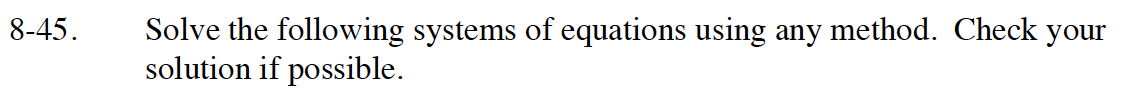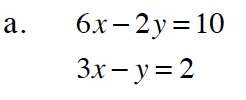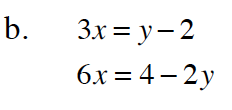### Home > INT1 > Chapter Ch8 > Lesson 8.1.3 > Problem8-45

8-45.
1. Solve the following systems of equations using any method. Check your solution if possible. Homework Help ✎

2.  a. 6x − 2y = 10 3x − y = 2 b. 3x = y − 2 6x = 4 − 2yRead the Math Notes boxes in Lesson 6.3.3 on Elimination and Lesson 6.4.1 on number of solutions in systems.

no solutionRead the Math Notes boxes in Lesson 6.3.3 on Elimination and Lesson 6.4.1 on number of solutions in systems.

The point of intersection (solution) for this system of equations is (0,2).